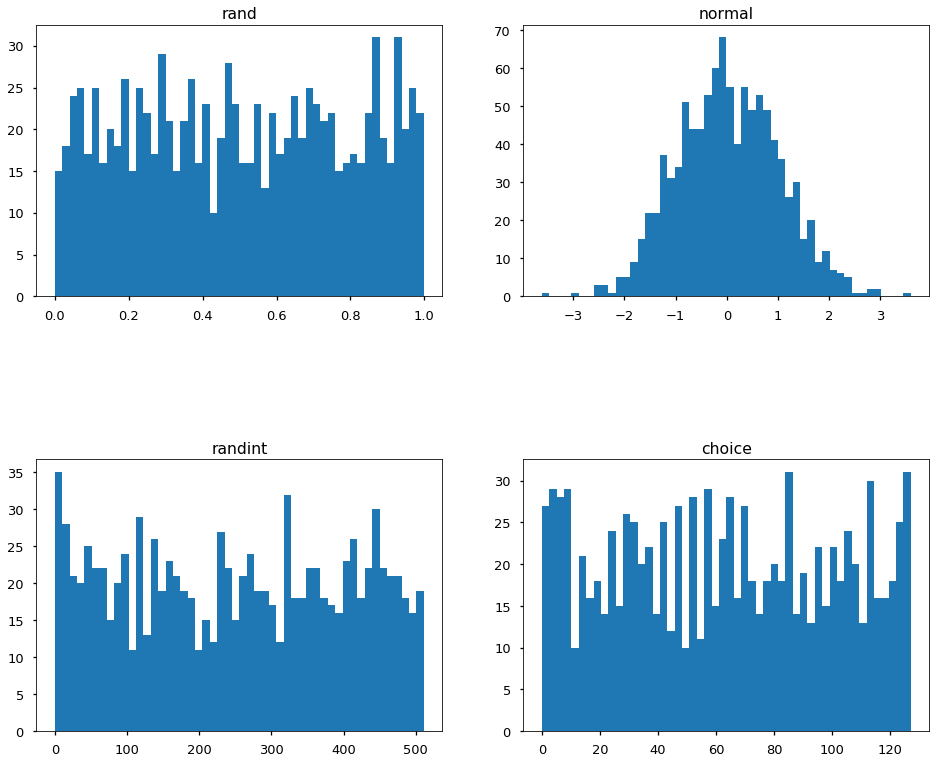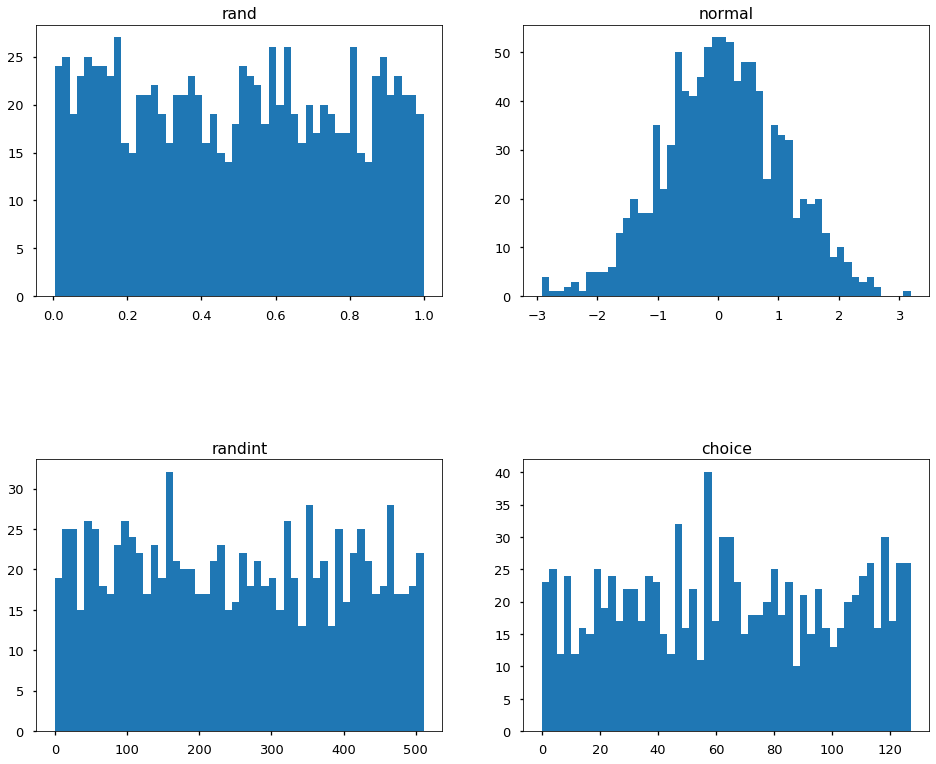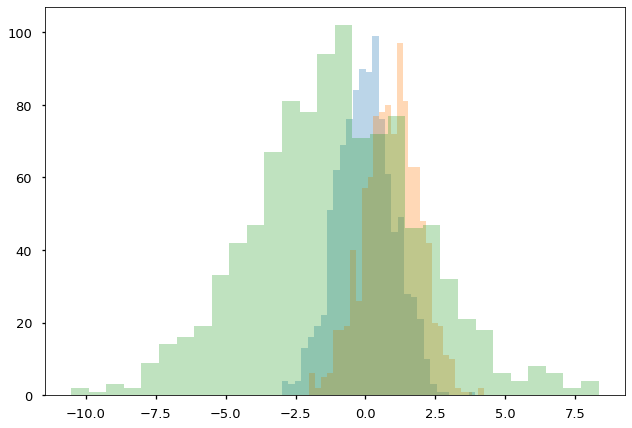02.02 Randomness in NumPy¶

The random module seem confusing at first due to the number of functions it provides. In reality you will be only using a handful of these functions.

We will get ahead of ourselves and use some plotting code. Please ignore it for now, we will see it shortly. For now we will use the plots to describe how the random numbers are generated.

Let's look at what kind of random numbers these functions generate.

In :
import numpy as np
%matplotlib inline
import matplotlib.pyplot as plt
plt.style.use('seaborn-talk')np-dice.svg

For a start we can use the np.random functions, these are good enough to generate some random numbers. The most common uses of randomness are here:

• rand generates floating point values between $0$ and $1$. taking them from a uniform distribution.

• normal takes random numbers from a normal distribution. Deep below the hood it uses rand and then a quantile function.

• randint is given two integers and it generates random integers between these values. Below the hood it uses rand.

• choice select values from the array given as the first argument at random. By default it does so with replacement but one can set replace=False in order to achieve choice without replacement. Below the hood it uses randint.

So, well, in the end the random module has just a single function that generates (pseudo-)random numbers. All other functions use the first random function and use its values in clever ways to achieve different and useful interfaces. On most computers even the first function which generates random values between $0$ and $1$ is just borrowed from the operating system.

Hence yes, all computers do is to generate random floating point numbers from a uniform distribution between $0$ and $1$. All other functions in a random library, including np.random are clever interfaces on how to turn those numbers between $0$ and $1$ into more convenient uses and distributions. And we can see the graphs of these distributions:

In :
r = {}
r['rand'] = np.random.rand(1024)
r['normal'] = np.random.normal(size=1024)
r['randint'] = np.random.randint(0, 512, 1024)
r['choice'] = np.random.choice(np.arange(128), 1024)

fig, ax = plt.subplots(2, 2, figsize=(16, 13))
order = ['rand', 'normal', 'randint', 'choice']
for idx, dist in enumerate(order):
ax[idx // 2, idx % 2].hist(r[dist], bins=50)
ax[idx // 2, idx % 2].title.set_text(dist)Note that as with array creation you can give a tuple as the shape of the resulting array returned by randomness generating functions. Or reshape it yourself afterward. As we have seen there is no significant difference since data is not copied.

Random numbers are all fun and good but sometimes we want number that are reasonably random but are always the same random numbers. What? Yes. We want random numbers that are known to us. This is useful if we have code that works reasonably well for most inputs and we want a known run of this code. Or to make a specific test of the results of such code.

We can seed the random number generator, this will give us a seeded object that works in a very similar way to np.random but which always outputs the same sequence of random numbers. If we change the seed he sequence changes but as long as the seed is the same the code will run in the same way every time. Here we replicate the above with the seed method:

In :
rng = np.random.RandomState(42)
r = {}
r['rand'] = rng.rand(1024)
r['normal'] = rng.normal(size=1024)
r['randint'] = rng.randint(0, 512, 1024)
r['choice'] = rng.choice(np.arange(128), 1024)

fig, ax = plt.subplots(2, 2, figsize=(16, 13))
order = ['rand', 'normal', 'randint', 'choice']
for idx, dist in enumerate(order):
ax[idx // 2, idx % 2].hist(r[dist], bins=50)
ax[idx // 2, idx % 2].title.set_text(dist)You may have noticed that we did call the normal procedure with a size= argument every time. This is because that function produces values from a normal distribution with specific parameters: the mean and standard deviation. By default the mean is $0$ and the standard deviation is $1$ but since these arguments come before the number of random numbers we want to retrieve from the distribution we need to explicitly name the argument above.

As you change the parameters of the normal distribution you generate random numbers with slightly different values. This is only visible if you generate a reasonably big amount of random numbers. Let's do exactly that: generate some random numbers from slightly different distributions:

In :
r = {}
r['n1'] = rng.normal(0, 1, size=1024)
r['n2'] = rng.normal(1, 1, size=1024)
r['n3'] = rng.normal(-1, 3, size=1024)
for dist in r.keys():
plt.hist(r[dist], bins=30, alpha=0.3)There are many more distributions to select random numbers from: binomial, pareto and weibull are some common ones. The full list of functions can be found in the numpy documentation. As well as in the in-built documentation.

In [ ]:
np.random?